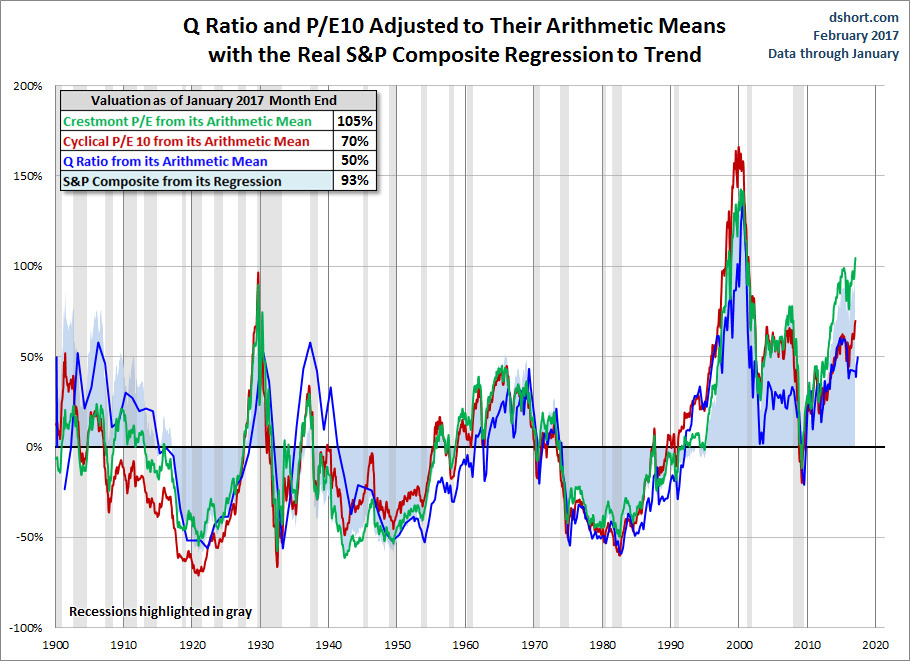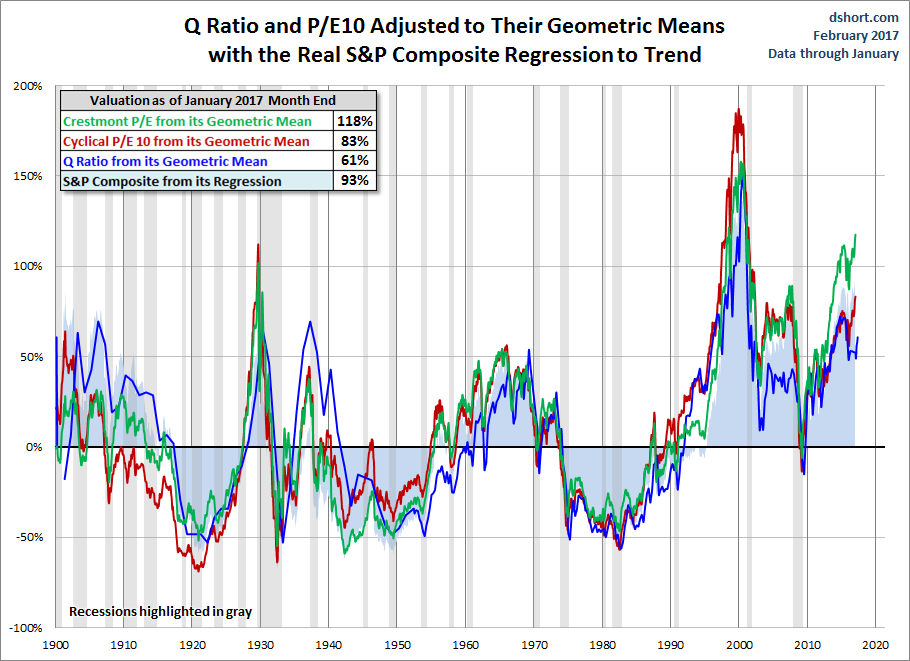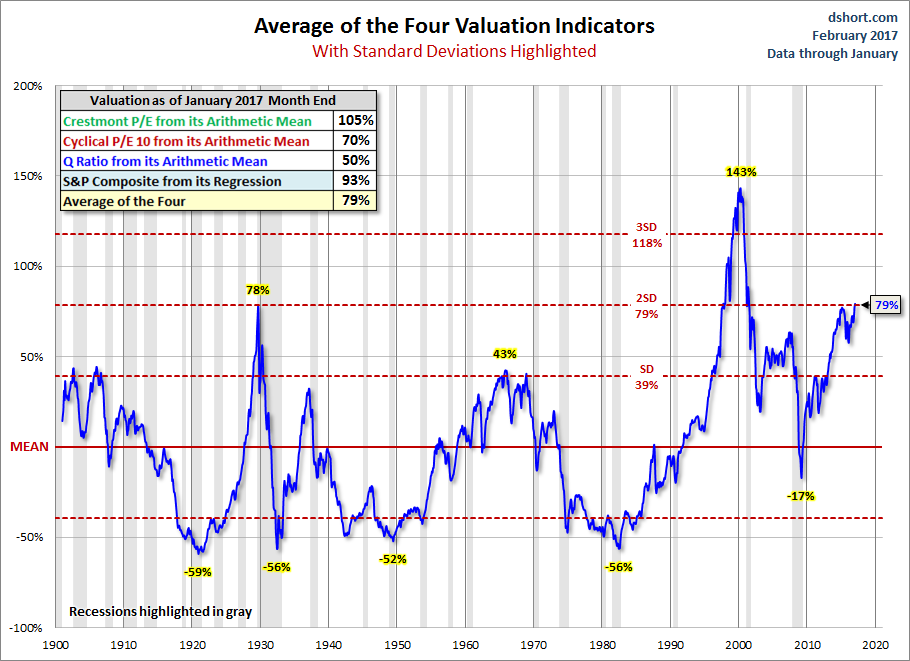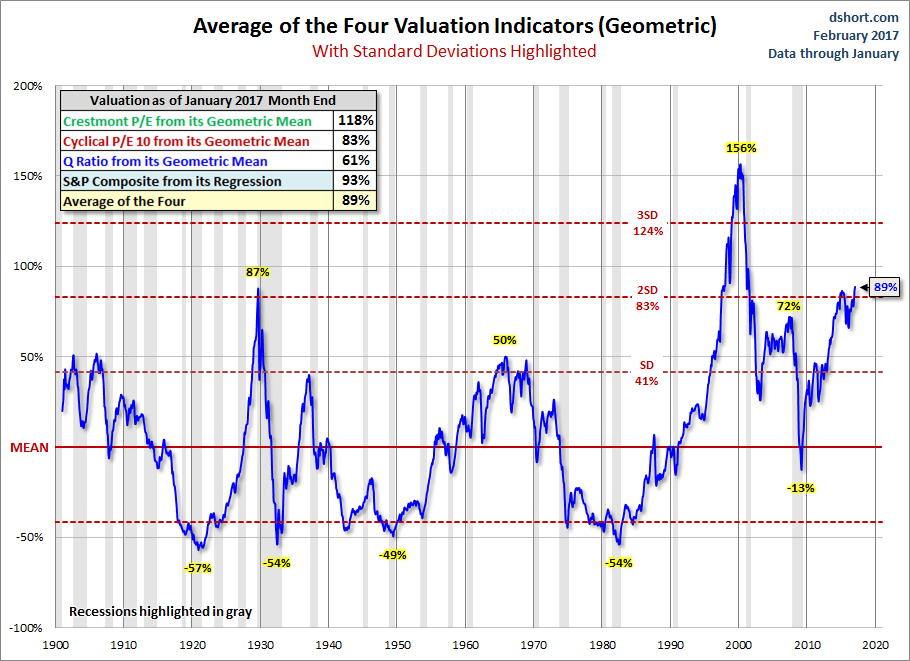# The S&P 500 Remains Historically OvervaluedFrom Jill Mislinski: As we head into the first full week of February, here is a summary of the four market valuation indicators we update on a monthly basis.

• The Crestmont Research P/E Ratio (more)
• The cyclical P/E ratio using the trailing 10-year earnings as the divisor (more)
• The Q Ratio, which is the total price of the market divided by its replacement cost (more)
• The relationship of the S&P Composite price to a regression trendline (more)

To facilitate comparisons, we’ve adjusted the two P/E ratios and Q Ratio to their arithmetic means and the inflation-adjusted S&P Composite to its exponential regression. Thus the percentages on the vertical axis show the over/undervaluation as a percent above mean value, which we’re using as a surrogate for fair value. Based on the latest S&P 500 monthly data, the market is overvalued somewhere in the range of 50% to 105%, depending on the indicator, up from the previous month’s 47% to 101%.

We’ve plotted the S&P regression data as an area chart type rather than a line to make the comparisons a bit easier to read. It also reinforces the difference between the line charts — which are simple ratios — and the regression series, which measures the distance from an exponential regression on a log chart.The chart below differs from the one above in that the two valuation ratios (P/E and Q) are adjusted to their geometric mean rather than their arithmetic mean (which is what most people think of as the “average”). The geometric mean increases our attention to outliers. In our view, the first chart does a satisfactory job of illustrating these four approaches to market valuation, but we’ve included the geometric variant as an interesting alternative view for the two P/Es and Q. In this chart, the range of overvaluation would be in the range of 61% to 118%, up from last month’s 58% to 114%.#### The Average of the Four Valuation Indicators

The next chart gives a simplified summary of valuations by plotting the average of the four arithmetic series (the first chart above) along with the standard deviations above and below the mean.

At the end of last month, the average of the four is 79% — reaching a new interim peak.Here is the same chart, this time with the geometric mean and deviations. The latest value of 89% also reached a new interim peak.As we’ve frequently pointed out, these indicators aren’t useful as short-term signals of market direction. Periods of over- and under-valuation can last for many years. But they can play a role in framing longer-term expectations of investment returns. At present, market overvaluation continues to suggest a cautious long-term outlook and guarded expectations. However, at today’s low annualized inflation rate and the extremely poor return on fixed income investments (Treasuries, CDs, etc.) the appeal of equities, despite overvaluation risk, is not surprising. For more on that topic, see our periodic update:

The SPDR S&P 500 ETF Trust (NYSE:SPY) closed at \$229.34 on Friday, up \$1.57 (+0.69%). Year-to-date, SPY has gained 2.60%, versus a % rise in the benchmark S&P 500 index during the same period.

SPY currently has an ETF Daily News SMART Grade of A (Strong Buy), and is ranked #1 of 109 ETFs in the Large Cap Blend ETFs category.